Free Shipping for Online Orders. Terms and conditions apply.

# Motor Sizing

Proper sizing and selection of a motor for your equipment is key to ensuring performance, reliability and cost of the equipment. In addition to the information below for properly sizing a motor, Oriental Motor offers online motor sizing tools as well as assistance from our Technical Support staff.

Our Technical Support Staff is available to assist you in properly sizing and selecting your motor based on your individual application. Simply call 1-800-GO-VEXTA (468-3982) (M-F 7:30am CST to 5:00pm PST).

## Selection Procedure

The first step is to determine the drive mechanism for your equipment. Some examples are direct rotation, a ball screw, a belt and pulley or a rack and pinion. Along with the type of drive mechanism, you must also determine the dimensions, mass and friction coefficient, etc. that are required for the load calculation:

• Dimensions and mass (or density) of load
• Dimensions and mass (or density) of each part
• Friction coefficient of the sliding surface of each moving part

Next you will need to determine the required specifications for the equipment:

• Operating speed and operating time
• Positioning distance and positioning time
• Resolution
• Stopping accuracy
• Position holding
• Power supply and voltage
• Operating environment
• Specific features and requirements such as; Open-Loop, Closed-Loop, Programmable, Feedback, IP rating, Agent approvals, etc.

In order to determine the performance you'll need from the motor, there are three factors to calculate; Moment of Inertia, Torque and Speed. (Refer to the below sections on calculations for each.)

Once you've calculated the inertia, torque and speed for the motor you'll select the type of motor based on the required specifications. Oriental Motor offers a wide range of stepper motors, servo motors, AC motors and brushless motors to meet the specific needs of your equipment.

Finally, after selecting the motor type you'll make a final determination of the motor by confirming the specifications of the selected motor (and gearhead if applicable) satisfy all of the requirements, such as mechanical strength, acceleration time and acceleration torque.

## Motor Sizing Calculations

There are three factors to calculate when sizing a motor; Moment of Inertia, Torque, and Speed.

### Moment of Inertia

Moment of inertia is the measure of an object's resistance to changes in its rotation rate.

When an object is just sitting without any motion, the moment of inertia is 0.

When you try to make it move that mean you want to change the speed of the object from 0 to any, there will be moment of inertia effect.

Fundamental Inertia (J) Equation: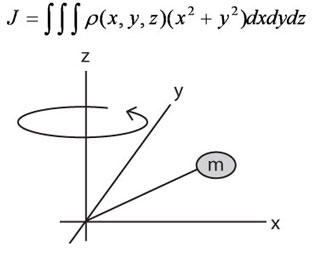Moment of Inertia Calculation for a Rotating Object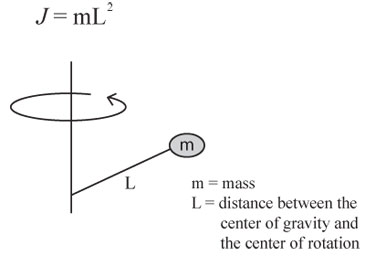Moment of Inertia Calculation for a Cylinder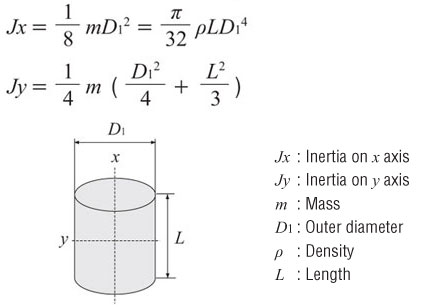Moment of Inertia Calculation for a Hollow Cylinder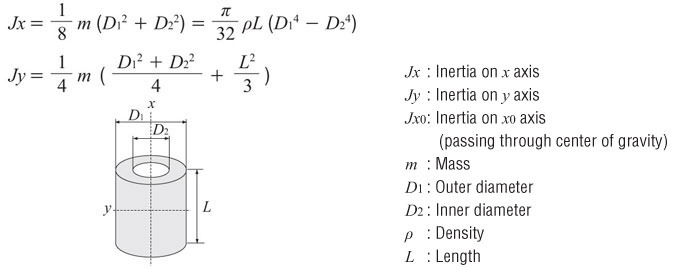Moment of Inertia Calculation for an Off-Center Axis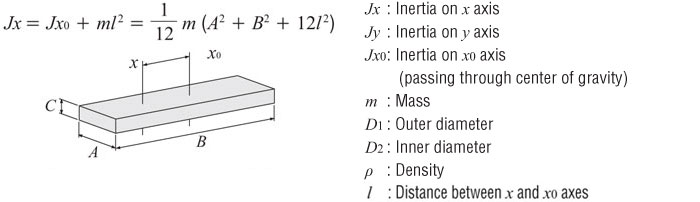Moment of Inertia Calculation for a Rectangular PillarMoment of Inertia Calculation for an Object in Linear Motion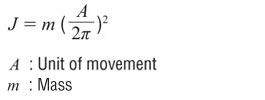### Units of Measure for Moment Inertia

The units of inertia are commonly used in two ways, oz-in² and oz-in-sec². The former includes gravity, the latter only mass.

Theoretically, inertia is factor of mass so it should not include gravity, however, practically we can not easily measure mass on the earth.

Oriental Motor commonly provides inertia in oz-in². Then, when we calculate the Acceleration Torque in Torque Calculation we divide the total the total inertia by the gravity from.

Gravity = 386 in/sec²

• oz-in² = Inertia based on weight
• oz-in-sec² = inertia based on mass

Calculation for oz-in² to oz-in-sec²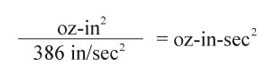## Torque

Torque is the tendency of a force to rotate an object about an axis. Torque is made up of two components; a load (constant) component and an acceleration component.

The load torque component is usually due to friction and/or gravity and is always acting on the motor. This component can usually be determined by calculation or by putting a torque wrench on the system and reading the torque value. When it is not able to measure, then we use some equations to calculate the approximate value.

The acceleration torque however, is only acting on the motor when it is accelerating or decelerating. Once the motor is running at a constant speed, this component goes away. Measuring the acceleration component is difficult not to mention dangerous. If you want the load to be up to speed within 50 milliseconds, it’s likely that a torque wrench will fly off. Therefore, we calculate the acceleration component. This component is a function of the inertia of the system and the acceleration rate. So, once we determine these values, we can figure out the acceleration torque.

As you see this equation torque is the product of the force and the distance between the force and the center of rotation. For example, if you want to hold the force acting on the end of pulley, T = F x r. So calculating load torque is determine the force in the system and the logical distance between the motor shaft and the where the force is acting.

When the mechanics become complicated, we need to convert the F and r to fit the mechanics.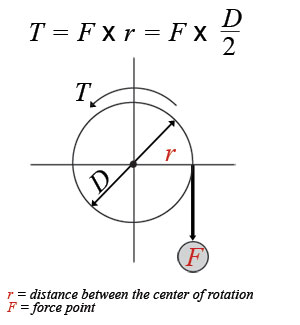If you can measure the force, that is the most accurate way to find the force since it takes care of the all efficiency and coefficient of frictions on the every part.

FB = Force when the main shaft begins to rotate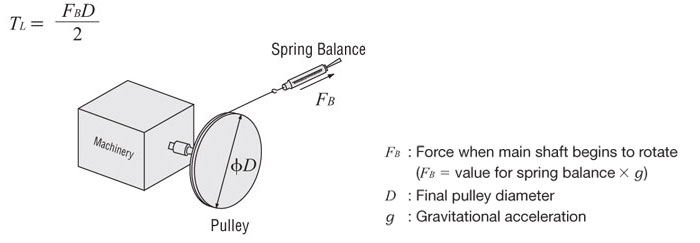### Forces

There are three types of forces; vertical, horizontal and incline. A force varies depending how it acts.

Vertical Force CalculationHorizontal Force CalculationIncline Force Calculation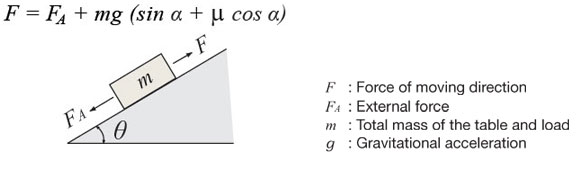Load Torque Calculation - Ball Screw DriveLoad Torque Calculation - Pulley Drive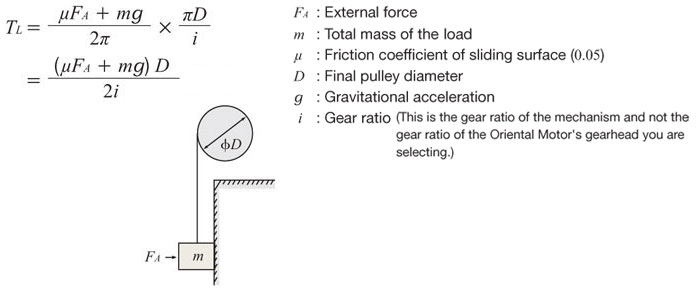Load Torque Calculation - Wire or Belt Drive, Rack and Pinion Drive### Acceleration Torque

As mentioned previously, acceleration torque is made up of inertia and acceleration rate. If we know those two values, we can calculate the acceleration torque.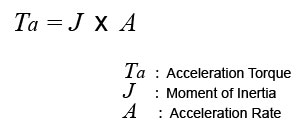Calculate the Acceleration Torque ( Ta )

If the motor speed is varied, the acceleration torque or deceleration torque must always be set.

The basic formula is the same for all motors. However, use the formulas below when calculating the acceleration torque for stepper or servo motors on the basis of pulse speed.

Common Formula for All MotorsWhen calculating the Acceleration Torque for Stepper or Servo Motors on the basis of pulse speed

There are two basic motion profiles. Acceleration/deceleration operation is the most common. When operating speed is low and load inertia is small, start/stop operation can be used.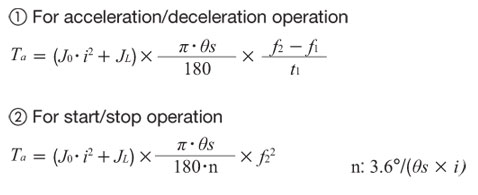Calculation for Required Torque ( TM )

The required torque is calculated by multiplying the sum of load torque and acceleration torque by the safety factor.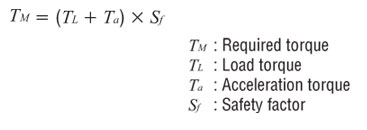Calculation for the Effective Load Torque ( Trms ) for Servo Motors and BX Series Brushless Motors

When the required torque for the motor varies over time, determine if the motor can be used by calculating the effective load torque. The effective load torque becomes particularly important for operating patterns such as fast-cycle operations where acceleration/deceleration is frequent. Calculate the effective load torque when selecting servo motors or the BX Series brushless motors.## Speed

Speed is determined by calculating the distance divided by time. For stepper or servo motors, acceleration time must also be accounted for.

Standard Speed Calculation

Speed = Distance / Time

For Stepper or Servo Motors

Speed = Distance / (Time - Acceleration Time ( t1 )Oriental Motor's Technical Support Team and Application Engineers will work with you to determine the best solution for your application. ORIENTAL MOTOR's experienced team members know the technology inside and out. We'll find the right solution based on your needs and explain the alternatives. Call 1-800-GO-VEXTA (468-3982) to speak with an Oriental Motor Technical Support Team Member.